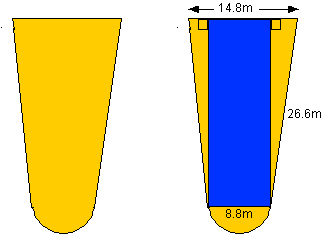+ Text Only Site
+ Non-Flash Version
+ Contact GlennBeginner's Guide to Aerodynamics Drag Equation - Level 2 Answers In Drag Equation Level 1, our pilot flew his first aircraft of the day. If you have not already done so, you may want to go to this activity first to prepare you for the second flight. Since the type of aircraft has changed, so has it's wing area. Once again, in order to work with our drag equation, we first need to find it's wing area. NOTE: Find the areas of each common figure and obtain their sum. You may assume the 2 right triangles are congruent.Work: Using the Pythagorean theorem, length of the rectangle = 26.4 m Total area = A(rectangle ) + 2 x A(triangle) + A(semicircle) Total area = 8.8 x 26.4 + 2 x 1/2 x 3 x 26.4 + 1/2 x 3.14 x 4.42 = 341.92 m2 for one wing 683.84 m2 for both wings The drag coefficient for our second aircraft was calculated at .365. When the pilot reached the desired cruise speed of 400 mph, the air density was .00052 slugs/cu. ft. What was the aircraft's thrust? NOTE: From the units table in Drag Equation Level 1, you must first convert the area to the proper units. (1 inch = 2.54 cm.) Unit Conversion: 1 ft = 30.48 cm = .3048 m. therefore, 3.28 ft = 1 m or 10.76 ft2 = 1m2 683.84 m2 x 10.76 ft2/m2 = 7358 ft2 Calculation: At cruise, F = D = Cd x r x V2/2 x A = .365 x .00052 x (4002 \2) x 7358 111,700 pounds of thrust The thrust of the first aircraft the pilot flew (Drag Equation - Level 1) under these same conditions is 150,000 pounds. If the 2 aircrafts weigh the same, which plane is more efficient? The second aircraft since the Lift to Drag ratio would be higher. (At cruise, Lift = Weight and Drag = Thrust)

Related Pages:
Standards
Activity
Worksheet
Aerodynamics Activity Index
Aerodynamics Index+ Inspector General Hotline + Equal Employment Opportunity Data Posted Pursuant to the No Fear Act + Budgets, Strategic Plans and Accountability Reports + Freedom of Information Act + The President's Management Agenda + NASA Privacy Statement, Disclaimer, and Accessibility CertificationEditor: Tom Benson NASA Official: Tom Benson Last Updated: Thu, May 13 02:38:39 PM EDT 2021 + Contact Glenn